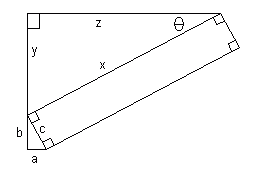I'm currently designing a garage and came upon this interesting math problem. I've tried using various methods to solve it but have so far been unsuccessful. I've included a picture as its far easier to show you my question than explain it verbally. I realize it could be done by trial and error but i'm looking for a real solution. Hi there. I've redrawn the diagram and added some extra labels for the sides that will help us. Notice that although it wasn't explicit in your diagram, I have assumed that the rectangular-looking part is indeed a rectangle (all angles are right angles).The first thing to notice is that the angle formed by sides c and b is congruent to theta. That means the two triangles are similar. Similar triangles have the property that the ratio of corresponding sides is equal. In our diagram, that means z/b = x/c. From your measurements, we know that b = 36.5 - y and from the pythagorean theorem, we know that y = sqrt(x2 - z2) and b = 36.5 - y. Let's substitute those into the ratios equation: z/(36.5 - sqrt(x2 - z2)) = x/c If you put the values z = 118.75 and c = 0.5 into this equation, you get 118.75/(36.5 - sqrt(x2 - 118.752)) = x/0.05, or 36.5x - 118.75/2 = x sqrt(x2 - 118.752) Squaring both sides and simplifying yields x4 + 15433.8 x2 - 4334.38 x + 3525.39 = 0 We used Mathematica to solve for x and got four solutions, only one of which makes sense for your situation. The solution is x = 124.09 feet. Since the cosine of the angle theta is z/x we used the inverse cosine function to find that theta is 0.2945 radians or 16.87 degrees. Stephen and Penny